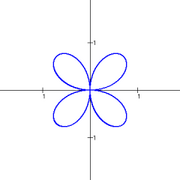A# rose curve

The rose curve is a curve that has the shape of a flower with petals. It was named rhodonea ("rose") by the Italian mathematician Guido Grandi in the 1720s. It is given by the polar equation

r = a sin().

If n is odd the rose has n petals, if n is even the rose has 2n petals, and if n = 2 the rose becomes the quadrifolium. If n is an irrational number, then there are an infinite number of petals.The quadrifolium: a special case of the rose curve